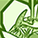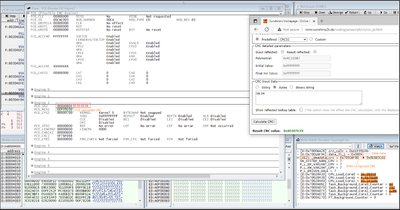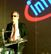# AURIX™ Forum DiscussionsLevel 3
Level 3# Calculate CRC-32 for 16bit data using FCE

Hi ,
Background : We transfer 12bit Single Channel ADC data over DMA and want to compute CRC using our SW and compare it with the checksum computed by the DMA. For our SW computation we use FCE to calculate CRC.
Problem :
Lets say the data we transferred by DMA = 0x804
DMA computed checksum = 0x8E6D5CEE

When I use FCE to compute the CRC the Input data is always taken in 32 bit format i.e 0x00 0x00 0x08 0x04.
And the CRC I get for this is 0xEEF0910D

Both the CRC's are correct the only difference is the input data format.
Question : Is it possible to use FCE to calculate CRC-32 for a 16 bit data ? If so could you please assist me on this.
Screenshot :Note : Changed DMA data transfer width to 32 bit and CRC computation is okay. But as we use only one ADC channel that other half contains some garbage data.

Thanks,
Kumaresh
5 RepliesModeratorModerator# Re: Calculate CRC-32 for 16bit data using FCE

Hi,

In FCE, the bit width of each word should be in terms of KERNEL polynomial width.
You would need to pad the 16-bit data.

https://community.infineon.com/t5/AURIX/Calculated-CRC-from-FCE-on-the-DMA-data-being-transferred-do...

Regards,
Aiswarya.Level 3
Level 3# Re: Calculate CRC-32 for 16bit data using FCE

Hi, If I pad other 16 bits to the data which I need to calculate CRC using FCE then it will be different by the CRC calculated by DMA.  Does the padded bits also considered in CRC calculation? From my testing I see that the CRC is computed with padded bits. So thought to confirm with youEmployee
Employee# Re: Calculate CRC-32 for 16bit data using FCE

Hi Kumaresh.  Is this for TC2xx?  If so, the incorrect value in DMA RDCRC for 8- and 16-bit transfers is a known issue - see DMA_TC.039 in the errata sheet.  The erratum was fixed in TC3xx.

The Read Data CRC (RDCRC) calculates an IEEE 802.3 ethernet CRC32 checksum as DMA moves read data through the DMA. The DMA implementation of the algorithm does not zero extend the read data for SDTB (8-bit) and SDTH (16-bit) accesses resulting in the calculation of a wrong checksum value.

The RDCRC must only be used with STDW (32-bit), SDTD (64-bit), BTR2 (128-bit) and BTR4 (256-bit) access sizes. It must be noted that SDTD, BTR2 and BTR4 are only supported for SRI-source to SRI-destination transactions.Level 3
Level 3# Re: Calculate CRC-32 for 16bit data using FCE

Hi, I observe this behaviour in TC377ModeratorModerator# Re: Calculate CRC-32 for 16bit data using FCE

Hello,

Could you please share the complete data stream fed to DMA and the FCE engine?

Kind Regards,
Aiswarya.